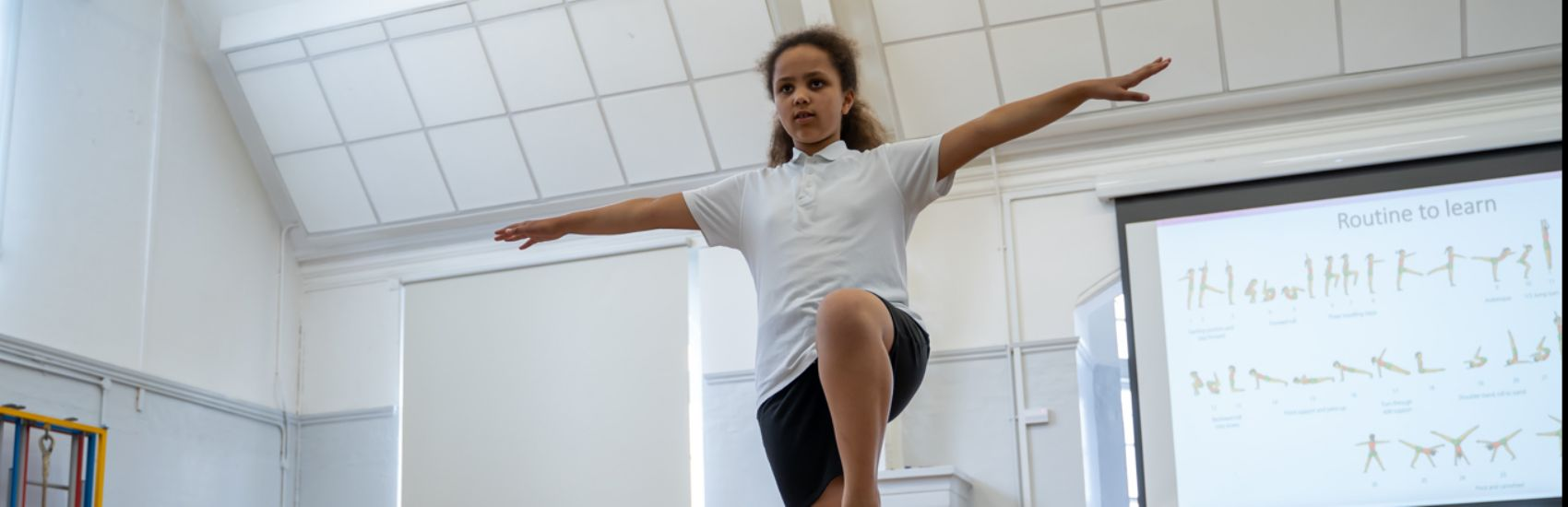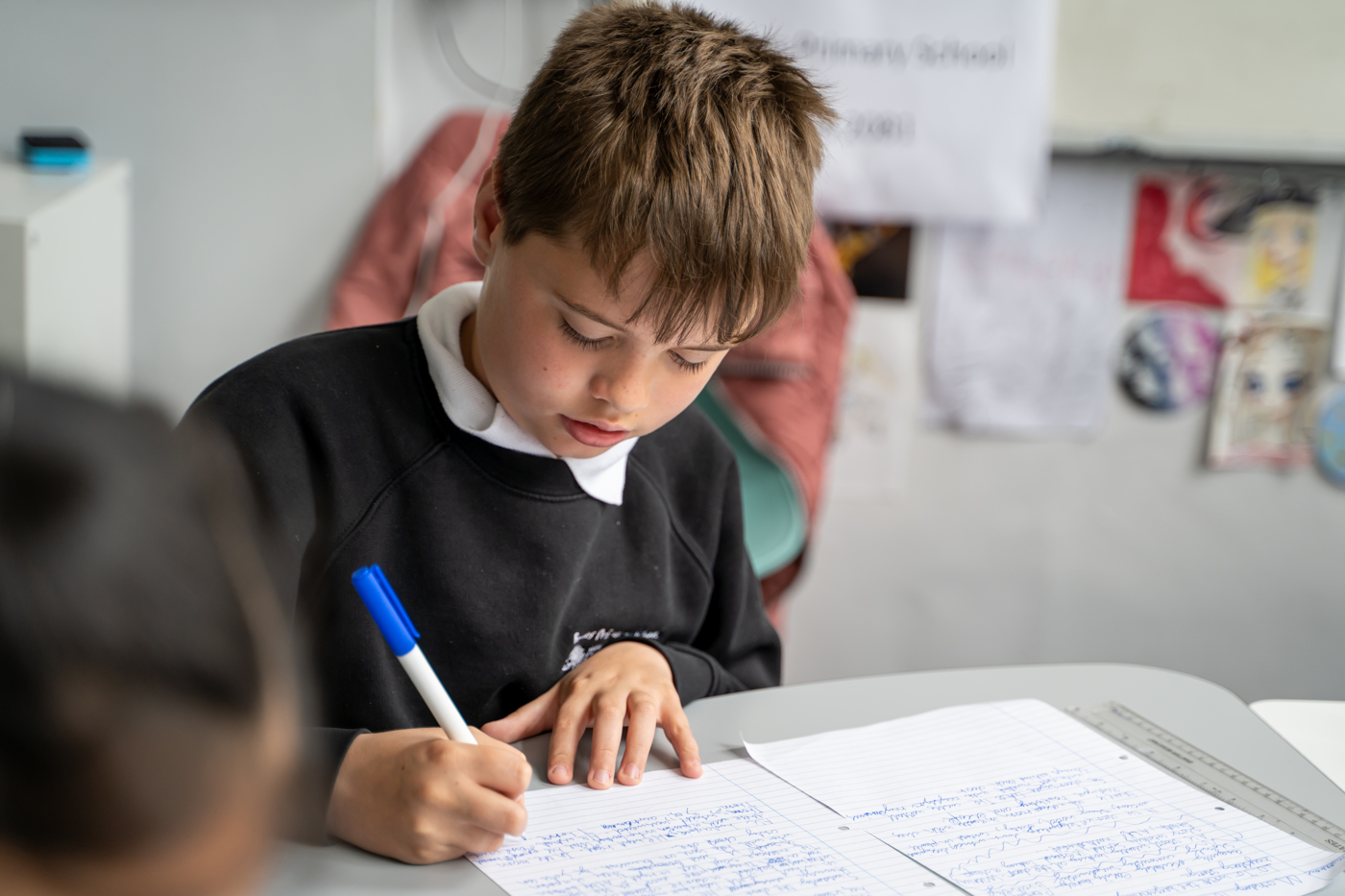''

# Mathematics

Mathematics National Curriculum for Year 4.

Number - number and place value
The children will be taught to:
• count in multiples of 6, 7, 9, 25 and 1000
• find 1000 more or less than a given number
• count backwards through zero to include negative numbers
• recognise the place value of each digit in a four-digit number (thousands, hundreds, tens, and ones)
• order and compare numbers beyond 1000
• identify, represent and estimate numbers using different representations
• round any number to the nearest 10, 100 or 1000
• solve number and practical problems that involve all of the above and with increasingly large positive numbers
• read Roman numerals to 100 (I to C) and know that over time, the numeral system changed to include the concept of zero and place value.
The children will be taught to:
• add and subtract numbers with up to 4 digits using the formal written methods of columnar addition and subtraction where appropriate
• estimate and use inverse operations to check answers to a calculation
• solve addition and subtraction two-step problems in contexts, deciding which operations and methods to use and why.
Number - multiplication and division
The children will be taught to:
• recall multiplication and division facts for multiplication tables up to 12 × 12
• use place value, known and derived facts to multiply and divide mentally, including: multiplying by 0 and 1; dividing by 1; multiplying together three numbers
• recognise and use factor pairs and commutativity in mental calculations
• multiply two-digit and three-digit numbers by a one-digit number using formal written layout
• solve problems involving multiplying and adding, including using the distributive law to multiply two digit numbers by one digit, integer scaling problems and harder correspondence problems such as n objects are connected to m objects.
Number - fractions (including decimals)
The children will be taught to:
• recognise and show, using diagrams, families of common equivalent fractions
• count up and down in hundredths; recognise that hundredths arise when dividing an object by one hundred and dividing tenths by ten.
• solve problems involving increasingly harder fractions to calculate quantities, and fractions to divide quantities, including non-unit fractions where the answer is a whole number
• add and subtract fractions with the same denominator
• recognise and write decimal equivalents of any number of tenths or hundredths
• recognise and write decimal equivalents to 1/4, 1/2, 3/4
• find the effect of dividing a one- or two-digit number by 10 and 100, identifying the value of the digits in the answer as ones, tenths and hundredths
• round decimals with one decimal place to the nearest whole number
• compare numbers with the same number of decimal places up to two decimal places
• solve simple measure and money problems involving fractions and decimals to two decimal places.
Measurement
The children will be taught to:
• convert between different units of measure [for example, kilometre to metre; hour to minute]
• measure and calculate the perimeter of a rectilinear figure (including squares) in centimetres and metres
• find the area of rectilinear shapes by counting squares
• estimate, compare and calculate different measures, including money in pounds and pence
• read, write and convert time between analogue and digital 12- and 24-hour clocks
• solve problems involving converting from hours to minutes; minutes to seconds; years to months; weeks to days.
Geometry - properties of shapes
The children will be taught to:
• compare and classify geometric shapes, including quadrilaterals and triangles, based on their properties and sizes
• identify acute and obtuse angles and compare and order angles up to two right angles by size
• identify lines of symmetry in 2-D shapes presented in different orientations
• complete a simple symmetric figure with respect to a specific line of symmetry.
Geometry - position and direction
The children will be taught to:
• describe positions on a 2-D grid as coordinates in the first quadrant
• describe movements between positions as translations of a given unit to the left/right and up/down
• plot specified points and draw sides to complete a given polygon.
Statistics
The children will be taught to:
• interpret and present discrete and continuous data using appropriate graphical methods, including bar charts and time graphs.
• solve comparison, sum and difference problems using information presented in bar charts, pictograms, tables and other graphs.